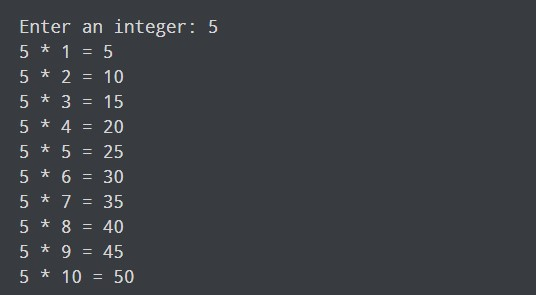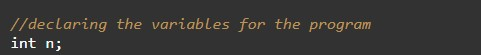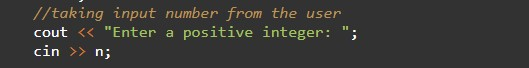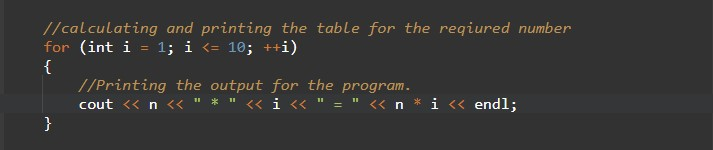# C++ Programs to Print Table of any Number

In this tutorial you will learn about the C++ Programs to Print Table of any Number and its application with practical example.

## C++ Program to Print Table of any Number

In this tutorial, we will learn to create a C++ program that will Print a Table of any Number in C++ programming.

## Prerequisites

Before starting with this tutorial we assume that you are best aware of the following C++ programming topics:

• Operators in C++ Programming.
• Basic Input and Output function in C++ Programming.
• Basic C++ Programming.

## Program to Print Table of any Number:-

As we all know the integer, float, or double value in c++ programming. In c++ programming, we can take the input from the user in numbers format. In today’s program, we will take input in number format. Secondly, we will Print a Table of any number with the help of a small program. The numbers are the most useful terminology in any programing language. For number/arithmetic manipulation in c++ programming, there are many predefined functions available.

Integers are the most use-full data types in any programming language. The integer values are used with operators to perform the best operations in programming.

With the help of this program, we can take input and print the Table of any Number.

## Output:-In the above program, we have first initialized the required variable.• n = it will hold the input number value from the user.
• i  = it will hold the integer value.

Taking Input numbers from the user.Calculating the table of given numbers and Printing the output.In this tutorial we have learn about the C++ Programs to Print Table of any Number and its application with practical example. I hope you will like this tutorial.